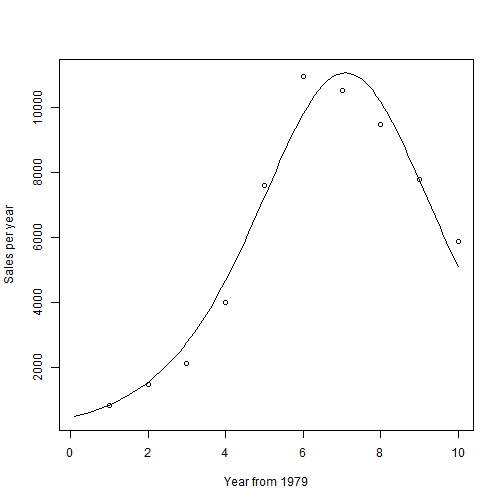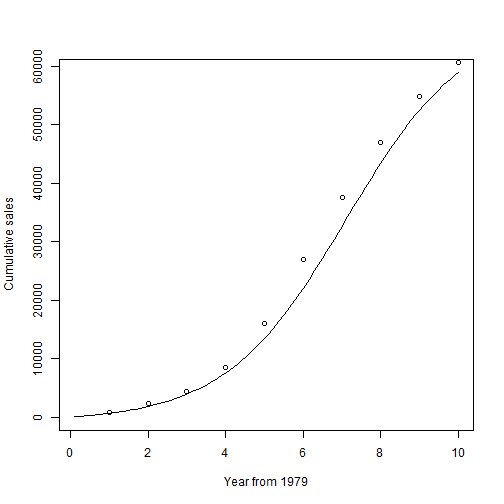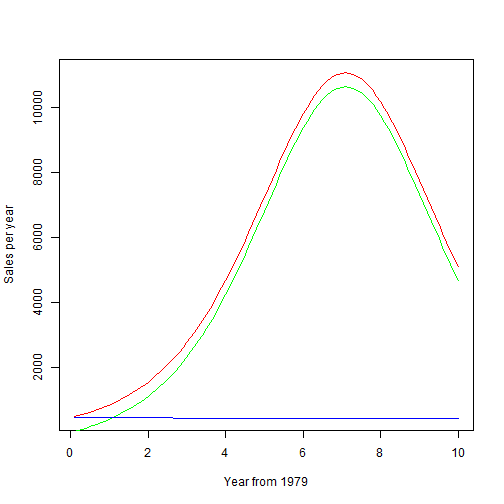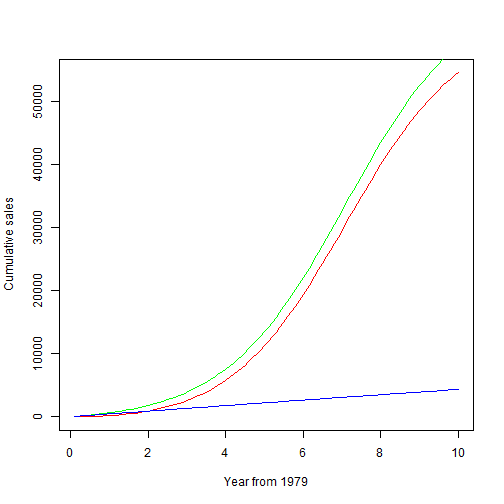# Title

``````# basss diffusion model chengjun, 20120424@canberra

# refer to http://en.wikipedia.org/wiki/Bass_diffusion_model and
# http://book.douban.com/subject/4175572/discussion/45634092/

# BASS Diffusion Model three parameters: the total number of people who
# eventually buy the product, m; the coefficient of innovation, p; and the
# coefficient of imitation, q

# example
T79 <- 1:10
Tdelt <- (1:100)/10
Sales <- c(840, 1470, 2110, 4000, 7590, 10950, 10530, 9470, 7790, 5890)
Cusales <- cumsum(Sales)
Bass.nls <- nls(Sales ~ M * (((P + Q)^2/P) * exp(-(P + Q) * T79))/(1 + (Q/P) *
exp(-(P + Q) * T79))^2, start = list(M = 60630, P = 0.03, Q = 0.38))
summary(Bass.nls)
``````
``````##
## Formula: Sales ~ M * (((P + Q)^2/P) * exp(-(P + Q) * T79))/(1 + (Q/P) *
##     exp(-(P + Q) * T79))^2
##
## Parameters:
##   Estimate Std. Error t value Pr(>|t|)
## M 6.80e+04   3.13e+03   21.74  1.1e-07 ***
## P 6.59e-03   1.43e-03    4.61   0.0025 **
## Q 6.38e-01   4.14e-02   15.41  1.2e-06 ***
## ---
## Signif. codes:  0 '***' 0.001 '**' 0.01 '*' 0.05 '.' 0.1 ' ' 1
##
## Residual standard error: 727 on 7 degrees of freedom
##
## Number of iterations to convergence: 8
## Achieved convergence tolerance: 7.32e-06
``````
``````
# get coefficient
Bcoef <- coef(Bass.nls)
m <- Bcoef
p <- Bcoef
q <- Bcoef
# setting the starting value for M to the recorded total sales.
ngete <- exp(-(p + q) * Tdelt)

# plot pdf
Bpdf <- m * ((p + q)^2/p) * ngete/(1 + (q/p) * ngete)^2
plot(Tdelt, Bpdf, xlab = "Year from 1979", ylab = "Sales per year", type = "l")
points(T79, Sales)
````````````
# plot cdf
Bcdf <- m * (1 - ngete)/(1 + (q/p) * ngete)
plot(Tdelt, Bcdf, xlab = "Year from 1979", ylab = "Cumulative sales", type = "l")
points(T79, Cusales)
````````````
# when q=0, only Innovator without immitators.
Ipdf <- m * ((p + 0)^2/p) * exp(-(p + 0) * Tdelt)/(1 + (0/p) * exp(-(p + 0) *
Tdelt))^2
# plot(Tdelt, Ipdf, xlab = 'Year from 1979',ylab = 'Isales per year',
# type='l')
Impdf <- Bpdf - Ipdf
plot(Tdelt, Bpdf, xlab = "Year from 1979", ylab = "Sales per year", type = "l",
col = "red")
lines(Tdelt, Impdf, col = "green")
lines(Tdelt, Ipdf, col = "blue")
````````````
# when q=0, only Innovator without immitators.
Icdf <- m * (1 - exp(-(p + 0) * Tdelt))/(1 + (0/p) * exp(-(p + 0) * Tdelt))
# plot(Tdelt, Icdf, xlab = 'Year from 1979',ylab = 'ICumulative sales',
# type='l')
Imcdf <- m * (1 - ngete)/(1 + (q/p) * ngete) - Icdf
plot(Tdelt, Imcdf, xlab = "Year from 1979", ylab = "Cumulative sales", type = "l",
col = "red")
lines(Tdelt, Bcdf, col = "green")
lines(Tdelt, Icdf, col = "blue")
``````